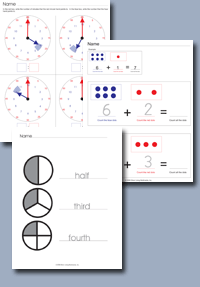﻿ Common Core Standards - Math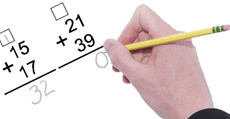Adaptive Worksheets

Helping Every Child to Succeed

toll-free (888) 777-0876
fax (888) 777-0875

## Graph points on the coordinate plane to solve real-world and mathematical problems

• CCSS.Math.Content.5.G.A.1 Use a pair of perpendicular number lines, called axes, to define a coordinate system, with the intersection of the lines (the origin) arranged to coincide with the 0 on each line and a given point in the plane located by using an ordered pair of numbers, called its coordinates. Understand that the first number indicates how far to travel from the origin in the direction of one axis, and the second number indicates how far to travel in the direction of the second axis, with the convention that the names of the two axes and the coordinates correspond (e.g., x-axis and x-coordinate, y-axis and y-coordinate).Find the Coordinates of a Point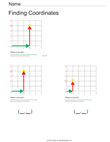Find the Coordinates of the Points
• CCSS.Math.Content.5.G.A.2 Represent real world and mathematical problems by graphing points in the first quadrant of the coordinate plane, and interpret coordinate values of points in the context of the situation.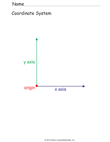Label the Parts of the Coordinate System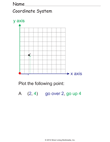Plot a Point on the Coordinate System

## Classify two dimensional figures into categories based on their properties

• CCSS.Math.Content.5.G.B.3 Understand that attributes belonging to a category of two-dimensional figures also belong to all subcategories of that category. For example, all rectangles have four right angles and squares are rectangles, so all squares have four right angles.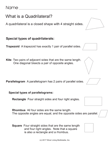Types of Quadrilaterals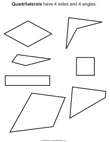Quadrilateral Examples
• CCSS.Math.Content.5.G.B.4 Classify two-dimensional figures in a hierarchy based on properties.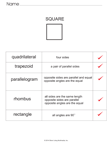Quadrilateral ChartQuadrilateral Hierarchy